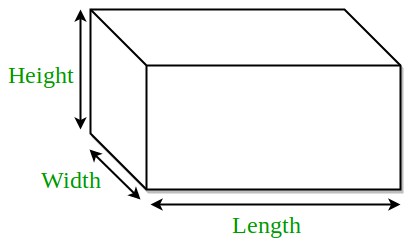GeeksforGeeks App
Open AppBrowser
Continue

# Program for Volume and Surface Area of Cuboid

Cuboid is a 3-dimensional box-like figure represented in the 3-dimensional plane.Cuboid has 6 rectangled-shape faces. Each face meet another face at 90 degree each.Three sides of cuboid meet at same vertex.Since it is made up of 6 rectangle faces, it have length, width and height of different dimension.Examples :

```Input : 2 3 4
Output : Area = 24
Total Surface Area = 52

Input : 5 6 12
Output : Area = 360
Total Surface Area = 324```

Formulae :

```Area = l*w*h
Total Surface Area = 2*l*w + 2*w*h + 2*l*h
where l, h, w are length, height and width of
cuboid respectively.```

## C++

 `// CPP program to find volume and``// total surface area of cuboid``#include ``using` `namespace` `std;`` ` `// utility function``double` `areaCuboid(``double` `l, ``double` `h, ``double` `w)``{``    ``return` `(l * h * w);``}`` ` `double` `surfaceAreaCuboid(``double` `l, ``double` `h, ``double` `w)``{``    ``return` `(2 * l * w + 2 * w * h + 2 * l * h);``}`` ` `// driver function``int` `main()``{``    ``double` `l = 1;``    ``double` `h = 5;``    ``double` `w = 7;``    ``cout << ``"Area = "` `<< areaCuboid(l, h, w) << endl;``    ``cout << ``"Total Surface Area = "` `         ``<< surfaceAreaCuboid(l, h, w);``    ``return` `0;``}`

## Java

 `// Java program to find volume and``// total surface area of cuboid`` ` `class` `GFG ``{``    ``// utility function``    ``static` `double` `areaCuboid(``double` `l, ``double` `h, ``                                           ``double` `w)``    ``{``        ``return` `(l * h * w);``    ``}`` ` `    ``static` `double` `surfaceAreaCuboid(``double` `l, ``double` `h, ``                                                ``double` `w)``    ``{``        ``return` `(``2` `* l * w + ``2` `* w * h + ``2` `* l * h);``    ``}``     ` `    ``// Driver code``    ``public` `static` `void` `main (String[] args) ``    ``{``        ``double` `l = ``1``;``        ``double` `h = ``5``;``        ``double` `w = ``7``;``        ``System.out.println(``"Area = "` `+ areaCuboid(l, h, w));``        ``System.out.println(``"Total Surface Area = "``                            ``+ surfaceAreaCuboid(l, h, w));``    ``}``}``// This code is contributed By Anant Agarwal.`

## Python3

 `# Python3 code to find volume and``# total surface area of cuboid`` ` `# utility function``def` `volumeCuboid( l , h , w ):``    ``return` `(l ``*` `h ``*` `w)``     ` `def` `surfaceAreaCuboid( l , h , w ):``    ``return` `(``2` `*` `l ``*` `w ``+` `2` `*` `w ``*` `h ``+` `2` `*` `l ``*` `h)`` ` `# driver function``l ``=` `1``h ``=` `5``w ``=` `7``print``(``"Volume ="` `, volumeCuboid(l, h, w))``print``(``"Total Surface Area ="``, surfaceAreaCuboid(l, h, w))`` ` `#This code is contributed by "Sharad_Bhardwaj".`

## C#

 `// C# program to find volume and``// total surface area of cuboid``using` `System;`` ` `class` `GFG {``     ` `    ``// utility function``    ``static` `double` `areaCuboid(``double` `l, ``double` `h,``                            ``double` `w)``    ``{``        ``return` `(l * h * w);``    ``}`` ` `    ``static` `double` `surfaceAreaCuboid(``double` `l, ``double` `h,``                                    ``double` `w)``    ``{``        ``return` `(2 * l * w + 2 * w * h + 2 * l * h);``    ``}`` ` `    ``// Driver code``    ``public` `static` `void` `Main()``    ``{``        ``double` `l = 1;``        ``double` `h = 5;``        ``double` `w = 7;``         ` `        ``Console.WriteLine(``"Area = "` `+ areaCuboid(l, h, w));``        ``Console.WriteLine(``"Total Surface Area = "``                        ``+ surfaceAreaCuboid(l, h, w));``    ``}``}`` ` `// This code is contributed By vt_m.`

## PHP

 ``

## Javascript

 ``

Output :

```Area = 35
Total Surface Area = 94```

Time complexity : O(1)
Auxiliary Space : O(1)

This article is contributed by Saloni Gupta . If you like GeeksforGeeks and would like to contribute, you can also write an article using write.geeksforgeeks.org or mail your article to review-team@geeksforgeeks.org. See your article appearing on the GeeksforGeeks main page and help other Geeks.